# NOT Function

Checks if one value is not equal to another

## What is the NOT Function?

The NOT function is an Excel Logical function. The function helps check if one value is not equal to another. If we give TRUE, it will return FALSE and when given FALSE, it will return TRUE. So, basically, it will always return a reverse logical value.

As a financial analyst, the NOT function is useful when we wish to know if a specific condition was not met.

### Formula

=NOT(logical)

Where:

1. Logical (required argument) – The argument should be a logical or numerical value. If the given logical argument is a numeric value, zero is treated as the logical value FALSE and any other numeric value is treated as the logical value TRUE.

### How to use the NOT Function in Excel?

NOT is a built-in function that can be used as a worksheet function in Excel. To understand the uses of this function, let us consider a few examples:

### Example 1

Suppose we don’t want the red and blue combination for soft toys. We are given the data below: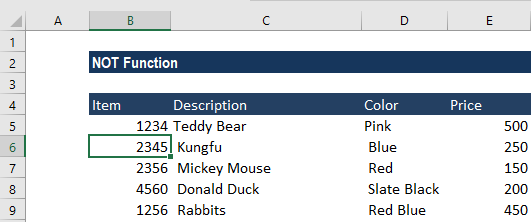To avoid the Red Blue combination, we will use the formula =NOT(C6=”Red Blue”).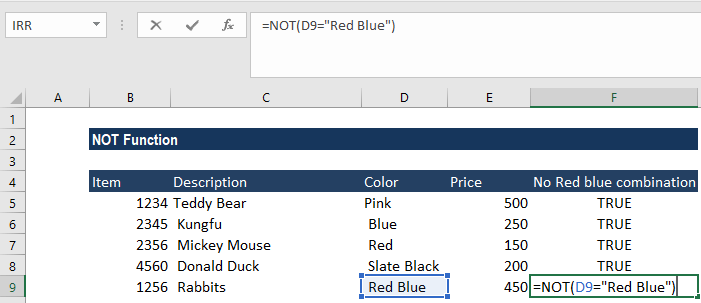We will get the results below: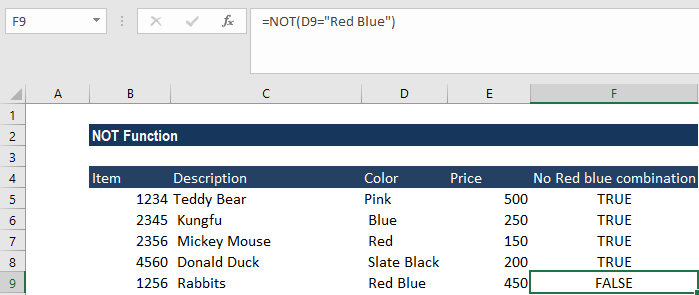If we wish to test several conditions in a single formula, then we can use NOT in conjunction with the AND or OR function. For example, if we wanted to exclude Red Blue and Slate Black, the formula would be =NOT(OR(C2=”Slate black”, C2=”Red Blue”).

### Example 2

Suppose we need to put “no bonus” for employees. Basically, we wish to reverse the behavior of some other functions. For instance, we can combine the NOT and ISBLANK functions to create the ISNOTBLANK formula.

The data given to us is shown below: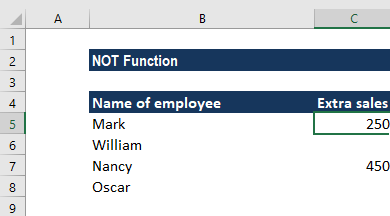The formula to be used would be =IF(NOT(ISBLANK(C5)), C5*0.25, “No bonus”), as shown below: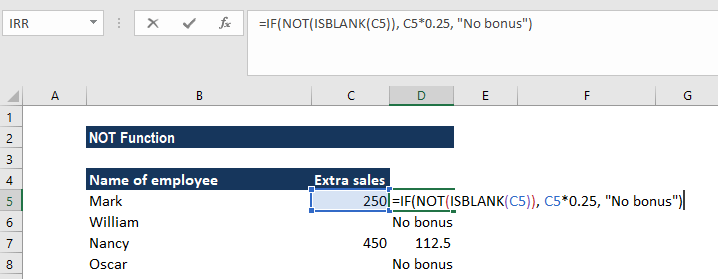The formula tells Excel to do the following:

• If the cell C5 is not empty, multiply the extra sales in C5 by 0.25, which gives the 25% bonus to each salesman who has made any extra sales.
• If there are no extra sales – that is, if C5 is blank – then the text, “No bonus”, appears.

We will get the results below: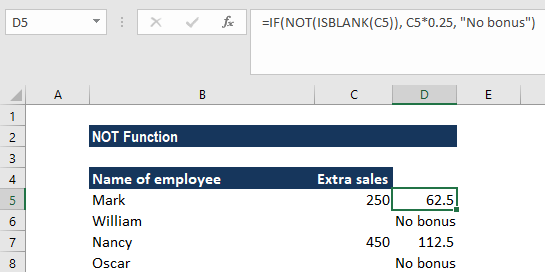In essence, this is how we use Logical functions in Excel. We can use this function with AND, XOR, and/or the OR function.

### Example 3

Let’s say we wish to highlight a cell that doesn’t meet specific criteria. In such a scenario, we can use NOT with the IF and OR function. Suppose we received an order to manufacture soft toys of Red and Blue color. The colors are specified by the client and we can’t change them.

We are given the inventory below: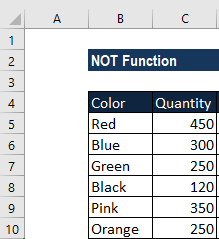Using the formula =IF(NOT(OR(B5=”red”,B5=”blue”)),”x”,””), we can highlight toys with the two colors: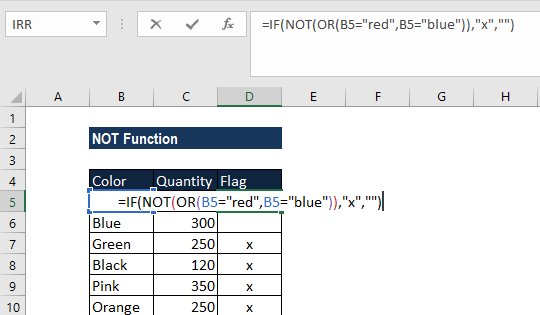We will get the following result: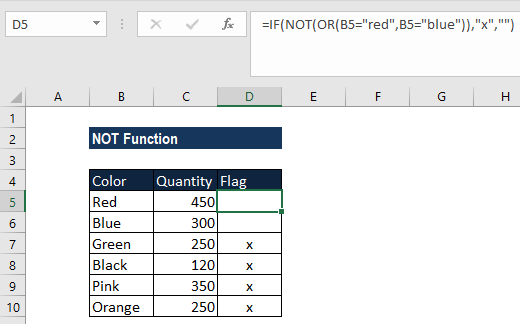Here, we marked inventory that was not of specific colors, that is, which were not Red or Blue.

Using NOT and OR, we will get TRUE if the specified cell is not Red or Blue.

We added an empty string “”  so if the result is FALSE, we get X, and nothing if TRUE. We can expand the OR function to add additional conditions if required.

### Things to remember

1. #VALUE! error – Occurs when the given argument is not a logical value or numeric value.

Thanks for reading CFI’s guide to important Excel functions! By taking the time to learn and master these functions, you’ll significantly speed up your financial analysis. To learn more, check out these additional CFI resources:

• Excel Functions for Finance